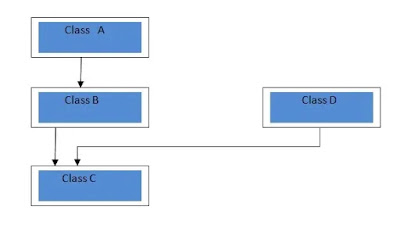Inheritance is a mechanism in which some or all the properties of parent is inherit to the child ( In real world).

Inheritance is a mechanism in which  there is a base class and derived class, base class is same as parent and derived class is same as child here derived class  get the property of the base class or base class inherit there property to the derived class. Inheritance is very useful because of there code reusability,

There are different types of inheritance:

1. Single inheritance
2. Multiple inheritance
3. Hierarchical inheritance
4. Multilevel inheritance
5.  Hybrid inheritance

Single inheritance:

In single inheritance  there is a   base class and derived class ,base class Inherit there property to the derived class

Multiple inheritance:

In multiple inheritance there are  numbers of base classes which Inherit there property to a single derived class. It is just like a child Inheriting the physical appearance of one parent and the intelligence of another.

The syntax of a derived class with multiple base classes:

Class D: visibility b, visibility b1.....
{…………………
……………………… (body of D)
};

Example

Class P: public M,public N

{

Public:

Void display (void);

};

Classes M and N have been specified:

Class M

{Protected:

int m;

Public:

Void get _m(int);
};

Class N
{
Protected:
int n;
Public :
Void get _n(int)
}

Hierarchical inheritance:

In hierarchical inheritance one is base class and other are derived class from one base class number of derived classes are formed.

Syntax of Hierarchical inheritance:

Class base_baseclassname

{

Visibility mode;

functions;

}

Class derived class: visibility mode baseclass

{

Visibility;

Functions;

}

Class derived class1 : visibility mode base_class

{

Visibility mode;

Functions;

}

Program to implement hierarchical inheritance

#include <iostream>

class A //single base class

{

public:

int x, y;

void getdata()

{

cout << "\nEnter value of x and y:\n"; cin >> x >> y;

}

};

class B : public A //B is derived from class base

{

public:

void product()

{

cout << "\nProduct= " << x * y;

}

};

class C : public A //C is also derived from base class

{

public:

void sum()

{

cout << "\nSum= " << x + y;

}

};

int main()

{

B obj1;          //object of derived class B

C obj2;          //object of derived class C

obj1.getdata();

obj1.product();

obj2.getdata();

obj2.sum();

return 0;

Multilevel inheritance:

Here A is the base class for the B class which is derived from the base class A and B is the base class for the derived class , In the given figure A is the base class ,B is the intermediate class and C is the derived class.

A derived class with multilevel inheritance is declared as follows:

Class A{……….};        //base class

Class B:public A{…………};   //B derived from A

Class C:public B{………….};   // C derived from B

Program to implement Multilevel inheritance.

#include <iostream.h>

class A

{

public:

void display()

{

cout<<"Base class content.";

}

};

class B : public A

{

};

class C : public B

{

};

void main()

{    C obj;

obj.display();

}

Hybrid inheritanceHybrid inheritance is a combination of two or more types of inheritance, Here in the given figure there are two different  types of inheritance is used to make a hybrid inheritance that is single inheritance and multilevel inheritance

Hybrid inheritance Syntax:-

class A

{

.........

};

class B : public A

{

..........

} ;

class C

{

...........

};

class D : public B, public C

{

...........

};

Program to implement hybrid inheritance:-

#include <iostream.h>

class A

{ public:  // visibility mode

int x;   //declaration of x

};

class B : public A

{

public:   //visibility mode

B()      //constructor to initialize x in base class A

{

x = 10;

}

};

class C

{

public:   // visibility mode

int y;

C()   //constructor to initialize y

{

y = 4;

}

};

class D : public B, public C //D is derived from class B and class C

{ public:

void sum()

{

cout << "Sum= " << x + y;

}

};

int main()

{

D obj1;          //object of derived class D

obj1.sum();

return 0; // return type

}